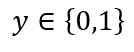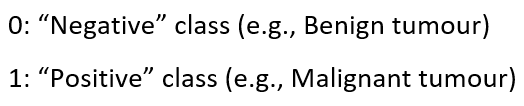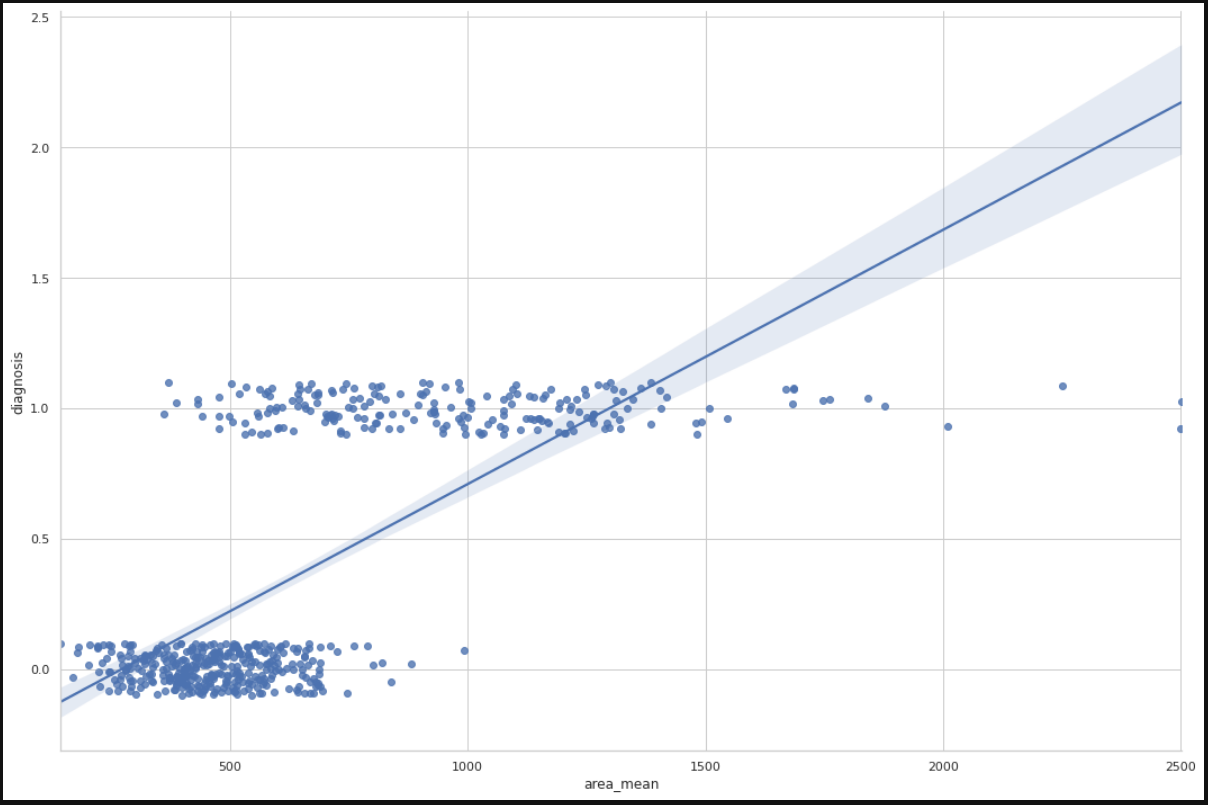1593293520

# Multiclass Classification With Logistic Regression

Logistic regression is a very popular machine learning technique. We use logistic regression when the dependent variable is categorical. This article will primarily focus on the implementation of logistic regression. I am assuming that you already know how to implement a binary classification with Logistic Regression. If not, please see the links at the end to learn the concepts of machine learning and the implementation of the basic logistic regression.

The implementation of Multiclass classification follows the same ideas as the binary classification. As you know in binary classification, we replace two classes with 1 and 0 respectively. In one vs all method, when we work with a class, that class is denoted by 1 and the rest of the classes becomes 0. It will be more understandable to you when you will implement it. I suggest, you keep coding and running the codes as you read.

## Python Implementation

Here I am going to show the implementation step by step.

1. Import the necessary packages and the dataset. I took the dataset from Andrew Ng’s Machine Learning course in Coursera. This is a handwriting recognition dataset. There are digits from 1 to 10. From the dataset of pixels, we need to recognize the digit.
``````import pandas as pd
import numpy as np
xl = pd.ExcelFile('ex3d1.xlsx')
y = pd.read_excel(xl, 'y', hearder = None)
``````

2. Define the hypothesis that takes the input variables and theta. It returns the calculated output variable.

``````def hypothesis(theta, X):
return 1 / (1 + np.exp(-(np.dot(theta, X.T)))) - 0.0000001
``````

3. Build the cost function that takes the input variables, output variable, and theta. It returns the cost of the hypothesis. That means it gives the idea about how far the prediction is from the original outputs.

``````def cost(X, y, theta):
y1 = hypothesis(X, theta)
return -(1/len(X)) * np.sum(y*np.log(y1) + (1-y)*np.log(1-y1))
``````

#data-science #multiclass-classification #logistic-regression #programming #machine-learning

## Buddha Community1593293520

## Multiclass Classification With Logistic Regression

Logistic regression is a very popular machine learning technique. We use logistic regression when the dependent variable is categorical. This article will primarily focus on the implementation of logistic regression. I am assuming that you already know how to implement a binary classification with Logistic Regression. If not, please see the links at the end to learn the concepts of machine learning and the implementation of the basic logistic regression.

The implementation of Multiclass classification follows the same ideas as the binary classification. As you know in binary classification, we replace two classes with 1 and 0 respectively. In one vs all method, when we work with a class, that class is denoted by 1 and the rest of the classes becomes 0. It will be more understandable to you when you will implement it. I suggest, you keep coding and running the codes as you read.

## Python Implementation

Here I am going to show the implementation step by step.

1. Import the necessary packages and the dataset. I took the dataset from Andrew Ng’s Machine Learning course in Coursera. This is a handwriting recognition dataset. There are digits from 1 to 10. From the dataset of pixels, we need to recognize the digit.
``````import pandas as pd
import numpy as np
xl = pd.ExcelFile('ex3d1.xlsx')
y = pd.read_excel(xl, 'y', hearder = None)
``````

2. Define the hypothesis that takes the input variables and theta. It returns the calculated output variable.

``````def hypothesis(theta, X):
return 1 / (1 + np.exp(-(np.dot(theta, X.T)))) - 0.0000001
``````

3. Build the cost function that takes the input variables, output variable, and theta. It returns the cost of the hypothesis. That means it gives the idea about how far the prediction is from the original outputs.

``````def cost(X, y, theta):
y1 = hypothesis(X, theta)
return -(1/len(X)) * np.sum(y*np.log(y1) + (1-y)*np.log(1-y1))
``````

#data-science #multiclass-classification #logistic-regression #programming #machine-learning1622617140

## Logistic Regression -Beginners Guide in Python - Analytics India Magazine

Most of the supervised learning problems in machine learning are classification problems. Classification is the task of assigning a data point with a suitable class. Suppose a pet classification problem. If we input certain features, the machine learning model will tell us whether the given features belong to a cat or a dog. Cat and dog are the two classes here. One may be numerically represented by 0 and the other by 1. This is specifically called a binary classification problem. If there are more than two classes, the problem is termed a multi-class classification problem. This machine learning task comes under supervised learning because both the features and corresponding class are provided as input to the model during training. During testing or production, the model predicts the class given the features of a data point.

This article discusses Logistic Regression and the math behind it with a practical example and Python codes. Logistic regression is one of the fundamental algorithms meant for classification. Logistic regression is meant exclusively for binary classification problems. Nevertheless, multi-class classification can also be performed with this algorithm with some modifications.

#developers corner #binary classification #classification #logistic regression #logit #python #regression #scikit learn #sklearn #statsmodels #tutorial1597019820

## Introduction:

In this article, I will be explaining how to use the concept of regression, in specific logistic regression to the problems involving classification. Classification problems are everywhere around us, the classic ones would include mail classification, weather classification, etc. All these data, if needed can be used to train a Logistic regression model to predict the class of any future example.

## Context:

1. Introduction to classification problems.
2. Logistic regression and all its properties such as hypothesis, decision boundary, cost, cost function, gradient descent, and its necessary analysis.
3. Developing a logistic regression model from scratch using python, pandas, matplotlib, and seaborn and training it on the Breast cancer dataset.
4. Training an in-built Logistic regression model from sklearn using the Breast cancer dataset to verify the previous model.

## Introduction to classification problems:

Classification problems can be explained based on the Breast Cancer dataset where there are two types of tumors (Benign and Malignant). It can be represented as:whereThis is a classification problem with 2 classes, 0 & 1. Generally, the classification problems have multiple classes say, 0,1,2 and 3.

## Dataset:

### Breast Cancer Wisconsin (Diagnostic) Data Set

Predict whether the cancer is benign or malignant

www.kaggle.com

1. Let’s import the dataset to a pandas dataframe:
``````import pandas as pd
``````

2. The following dataframe is obtained:

``````df.head()

![Image for post](https://miro.medium.com/max/1750/1*PPyiGgocvjHbgIcs9yTWTA.png)

``````

df.info()

### Data analysis:

Let us plot the mean area of the clump and its classification and see if we can find a relation between them.

``````import matplotlib.pyplot as plt
import seaborn as sns
from sklearn import preprocessing
label_encoder = preprocessing.LabelEncoder()
df.diagnosis = label_encoder.fit_transform(df.diagnosis)
sns.set(style = 'whitegrid')
sns.lmplot(x = 'area_mean', y = 'diagnosis', data = df, height = 10, aspect = 1.5, y_jitter = 0.1)
``````We can infer from the plot that most of the tumors having an area less than 500 are benign(represented by zero) and those having area more than 1000 are malignant(represented by 1). The tumors having a mean area between 500 to 1000 are both benign and malignant, therefore show that the classification depends on more factors other than mean area. A linear regression line is also plotted for further analysis.

#machine-learning #logistic-regression #regression #data-sceince #classification #deep learning1598352300

## Regression: Linear Regression

Machine learning algorithms are not your regular algorithms that we may be used to because they are often described by a combination of some complex statistics and mathematics. Since it is very important to understand the background of any algorithm you want to implement, this could pose a challenge to people with a non-mathematical background as the maths can sap your motivation by slowing you down.In this article, we would be discussing linear and logistic regression and some regression techniques assuming we all have heard or even learnt about the Linear model in Mathematics class at high school. Hopefully, at the end of the article, the concept would be clearer.

**Regression Analysis **is a statistical process for estimating the relationships between the dependent variables (say Y) and one or more independent variables or predictors (X). It explains the changes in the dependent variables with respect to changes in select predictors. Some major uses for regression analysis are in determining the strength of predictors, forecasting an effect, and trend forecasting. It finds the significant relationship between variables and the impact of predictors on dependent variables. In regression, we fit a curve/line (regression/best fit line) to the data points, such that the differences between the distances of data points from the curve/line are minimized.

#regression #machine-learning #beginner #logistic-regression #linear-regression #deep learning1593411000

## Logistic Regression: Discover the Powerful Classification Technique

Get to know one of the most widely used classification techniques
The process of differentiating categorical data using predictive techniques is called classification. On the basis of training data, consisting of observations whose category membership is known, the classifier (the algorithm that implements classification) should learn, on the basis of explanatory variables (features), to which category new observations belong.

#algorithms #classification #logistic-regression #machine-learning #data-science #algorithms